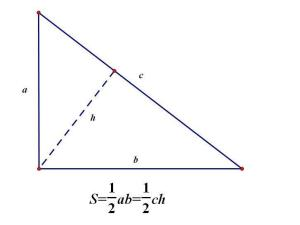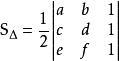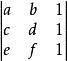# 三角形面积公式三角形面积公式：S=（底x高）÷2=(1/2)x底x高。

（1）三角形ABC的任何一条边都可以作底；（2）顶点到“底”的距离称为三角形的“高”。三角形面积公式1.已知三角形底a，高h，则S＝ah/2

2.已知三角形三边a,b,c，则s=1/4*√[2(a^2b^2+ a)(p - b)(p - c)] （海伦公式）（p=(a+b+c)/2）

S=sqrt[p(p-a)(p-b)(p-c)]

=sqrt[(1/16)(a+b+c)(a+b-c)(a+c-b)(b+c-a)]

=1/4sqrt[(a+b+c)(a+b-c)(a+c-b)(b+c-a)]

3.已知三角形两边a,b,这两边夹角C，则S＝absinC/2，即两夹边之积乘夹角的正弦值。

4.设三角形三边分别为a、b、c，内切圆半径为r

5.设三角形三边分别为a、b、c，外接圆半径为R

6.行列式形式7.海伦——秦九韶三角形中线面积公式:

S=√[(Ma+Mb+Mc)*(Mb+Mc-Ma)*(Mc+Ma-Mb)*(Ma+Mb-Mc)]/3

8.根据三角函数求面积：

S= &frac12;ab sinC=2R&sup2; sinAsinBsinC= a&sup2;sinBsinC/2sinA

9.根据向量求面积：

SΔ)= &frac12;√(|AB|*|AC|)&sup2;-（AB*AC）&sup2 .

10.在直角坐标系中，三角形ABC面积为

S=|AB×AC|/2

10.已知三角形三边a、b、c,则S＝ √{1/4[c^2a^2-((c^2+a^2-b^2)/2)^2]} (“三斜求积” 南宋秦九韶）

| a b 1 |

S△=1/2 * | c d 1 |

| e f 1 |

【| a b 1 |

| c d 1 | 为三阶行列式,此三角形ABC在平面直角坐标系内A(a,b),B(c,d), C(e,f),这里ABC

| e f 1 |